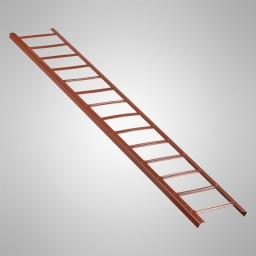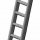# Right triangle

Ladder 16 feet reaches up 14 feet on a house wall. The 90-degree angle at the base of the house and wall. What are the other two angles or the length of the leg of the yard?

b =  7.746 ft
A =  61.045 °
B =  28.955 °

### Step-by-step explanation:Did you find an error or inaccuracy? Feel free to write us. Thank you!Tips to related online calculators

#### You need to know the following knowledge to solve this word math problem:

We encourage you to watch this tutorial video on this math problem:

## Related math problems and questions:

• 30-60-90The longer leg of a 30°-60°-90° triangle measures 5. What is the length of the shorter leg?The ladder touch on a wall at a height of 7.5 m. The angle of the inclination of the ladder is 76°. How far is the lower end of the ladder from the wall?The ladder has a length of 3 m and is leaning against the wall, and its inclination to the wall is 45°. How high does it reach?What is the slope of a ladder 6.2 m long and 5.12 m in height.
• Right triangleCalculate the missing side b and interior angles, perimeter, and area of ​​a right triangle if a=10 cm and hypotenuse c = 16 cm.
• Elevation anglesFrom the endpoints of the base 240 m long and inclined at an angle of 18° 15 ', the top of the mountain can be seen at elevation angles of 43° and 51°. How high is the mountain?
• Medians of isosceles triangleThe isosceles triangle has a base ABC |AB| = 16 cm and 10 cm long arm. What is the length of medians?
• ISO trapeziumCalculate area of isosceles trapezoid with base 95 long, leg 27 long and with the angle between the base and leg 70 degrees.
• Inner anglesThe inner angles of the triangle are 30°, 45° and 105° and its longest side is 10 cm. Calculate the length of the shortest side, write the result in cm up to two decimal places.
• Perimeter of triangleIn the triangle, ABC angle A is 60° angle B is 90°, and side size c is 15 cm. Calculate the triangle circumference.
• IS triangleCalculate interior angles of the isosceles triangle with base 40 cm and legs 22 cm long.
• A trapezoidA trapezoid with a base length of a = 36.6 cm, with angles α = 60°, β = 48° and the height of the trapezoid is 20 cm. Calculate the lengths of the other sides of the trapezoid.
• Right triangleCalculate the length of the remaining two sides and the angles in the rectangular triangle ABC if a = 10 cm, angle alpha = 18°40'.
• Isosceles triangle 10In an isosceles triangle, the equal sides are 2/3 of the length of the base. Determine the measure of the base angles.
• Michael 2Michael has a 35 foot ladder leaning against the side of his house. If the bottom of the ladder is 21 feet away from his house, how many feet above the ground does the ladder touch the house?
• TrianglesFind out whether given sizes of the angles can be interior angles of a triangle: a) 23°10',84°30',72°20' b) 90°,41°33',48°37' c) 14°51',90°,75°49' d) 58°58',59°59',60°3'
• CloudsApproximately at what height is the cloud we see under an angle of 26°10' and see the Sun at an angle of 29°15' and the shade of the cloud is 92 meters away from us?